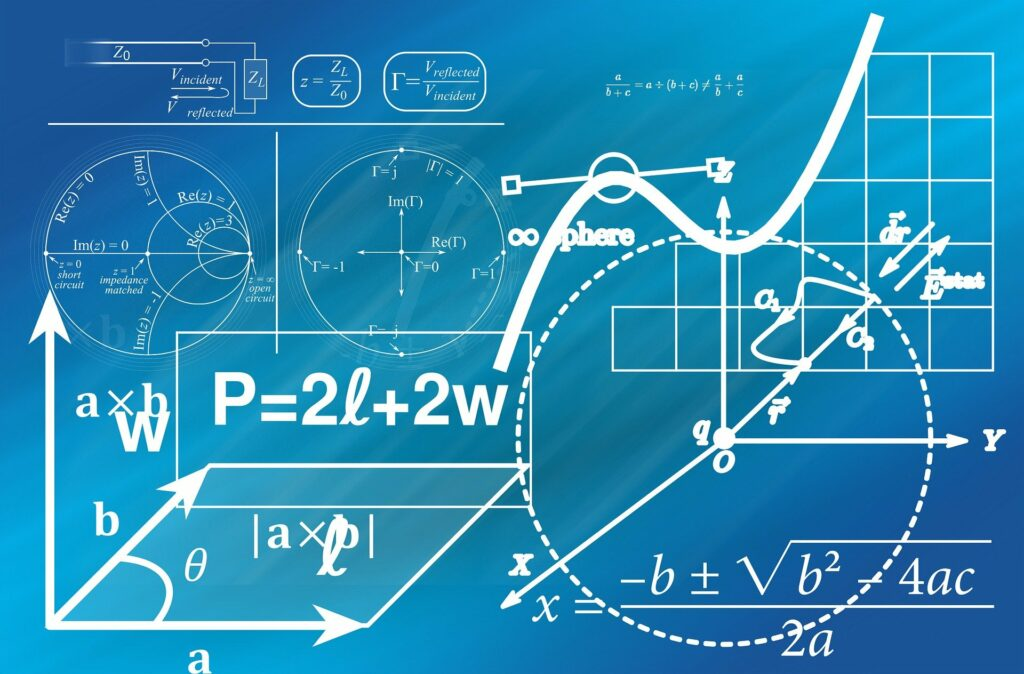# Exploring the Distance Between Two Points: A Mathematical Journey

Embark on a journey through the realms of mathematics and physics as we explore the intriguing concepts of distance and speed. From the simplicity of calculating the distance between two points to the practicality of determining speed, this article demystifies these fundamental concepts with easy-to-understand examples and calculations. Whether you’re a student, a professional, or just a curious mind, this exploration offers valuable insights into the world of motion and measurement. Join us as we unravel the mysteries of distance and speed, making these concepts accessible to everyone.

In the world of mathematics and physics, understanding the distance between two points is more than just a theoretical concept; it’s a practical tool used in various fields such as geography, astronomy, and engineering. This article delves into the simplicity and elegance of calculating this distance, a process that surprisingly requires only elementary math skills. Furthermore, we will explore how this concept is intricately linked to calculating speed, an essential aspect of motion in our daily lives.

#### The Essence of the Distance Formula

The distance formula is rooted in the Cartesian coordinate system, a fundamental component of geometry. This system, developed by René Descartes, uses two perpendicular axes (x and y) to define the position of points in a two-dimensional space. To calculate the distance between any two points in this space, such as (x1, y1) and (x2, y2), one can follow these simple steps:

1. Subtracting Coordinates: Find the difference between corresponding coordinates of the two points. This involves subtracting x-coordinates (x2 – x1) and y-coordinates (y2 – y1).
2. Squaring the Differences: Square each of these differences. This step eliminates any negative values and is crucial for the next step.
3. Adding the Squares: Add the squares of these differences together. This step is in line with the Pythagorean theorem, a cornerstone of geometry.
4. Square Root of the Sum: The final step is to take the square root of the sum obtained in the previous step. This value is the distance between the two points.

For those looking for an efficient way to perform these calculations, the distance formula calculator is an excellent resource.

#### Practical Applications of the Distance Formula

The distance formula finds its application in various real-world scenarios:

• Navigation and Mapping: It helps in calculating the shortest path between two locations, crucial for air and sea navigation.
• Astronomy: Astronomers use it to estimate the distances between celestial bodies.
• Architecture and Engineering: It’s used in designing and planning structures, ensuring precision and safety.
• Computer Graphics: In video games and simulations, this formula helps in rendering 3D objects and environments.

#### Speed Calculation: A Direct Offshoot

Once the distance is known, calculating the speed becomes a straightforward task. Speed, defined as the rate at which an object covers a distance, is calculated using the formula: Speed = Distance / Time. This formula is pivotal in various aspects of daily life and scientific study, from determining the speed of vehicles to understanding the motion of planets.

##### Types of Speed:
• Average Speed: This is the total distance traveled divided by the total time taken. It gives a general idea of the speed over a period.
• Instantaneous Speed: This refers to the speed of an object at a particular moment. It’s what we see on a car’s speedometer.

For a deeper understanding of these concepts, including practical examples, visit How to Calculate Distance and Speed.

#### Conclusion

The distance between two points might seem like a simple concept, but its applications are vast and varied. From helping sailors navigate the seas to enabling architects to design safer buildings, this fundamental concept of geometry plays a crucial role in our world. Similarly, understanding speed not only helps us in everyday tasks like driving but also in comprehending the motion of objects in the universe. Both these concepts, intertwined and essential, demonstrate the beauty and utility of mathematics in our daily lives.

Latest posts by Answer Prime (see all)
Scroll to Top